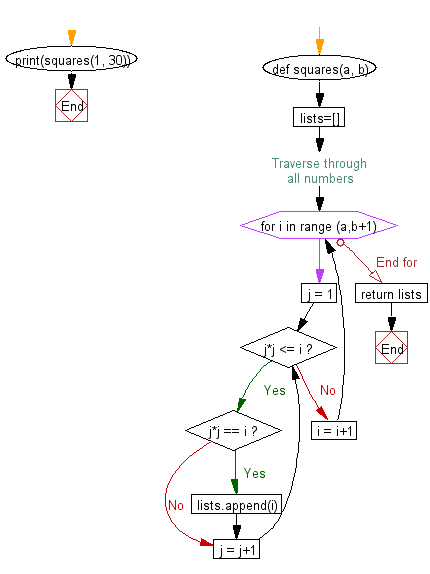﻿ Python Math: Find perfect squares between two given numbers - w3resource# Python Math: Find perfect squares between two given numbers

## Python Math: Exercise-78 with Solution

Write a Python program to find perfect squares between two given numbers.

Sample Solution:-

Python Code:

``````def squares(a, b):
lists=[]
# Traverse through all numbers
for i in range (a,b+1):
j = 1;
while j*j <= i:
if j*j == i:
lists.append(i)
j = j+1
i = i+1
return lists
print(squares(1, 30))
```
```

Sample Output:

```[1, 4, 9, 16, 25]
```

Flowchart:## Visualize Python code execution:

The following tool visualize what the computer is doing step-by-step as it executes the said program:

Python Code Editor:

Have another way to solve this solution? Contribute your code (and comments) through Disqus.

What is the difficulty level of this exercise?

Test your Programming skills with w3resource's quiz.

﻿

```>>> students = [{'name': 'John', 'score': 98}, {'name': 'Mike', 'score': 94}, {'name': 'Jennifer', 'score': 99}]